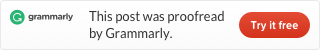# Heap and Heap-Sort algorithm (Java code and Leetcode problems)

Note: Heap in algorithm and data structure is a different concept with the heap in memory management. Heap in memory management is more like a pool. There is a discussion at StackOverflow, we don’t talk about the detail of this different. You know we are talking about the heap concept in algorithm and data structure is enough for us. We still follow the style of Introduction to Algorithms.

Heap is a complete binary tree represented by an array. A binary tree is one kind of tree, every parent node has at most two children, we call them left-subtree and right-subtree.

A perfect binary tree is a binary tree when the layer count is k, it has 2k-1 nodes. Like this:

And we can think a complete binary tree is a perfect binary tree leak or not lack some nodes but must fill the nodes from top to bottom, left to right. It means only can lack some nodes from the right. Like this:

When we use a heap to store this complete binary tree, we can save it as an array like this: [A, B, C, D, E, F, G, H, I, J, K, L]. Use this storage method, we will find out that the index of the root of this tree is 1.

And the index of every parent node is the children node’s index divide to 2. And left sub-node’s index is its parent node’s index multiply 2, right sub-node’s index is its parent node’s index multiply 2 then add 1.

So we get the function to access children and parent nodes.

Then we discuss a data structure, Max-Heap. It is a heap, every parent bigger than its children. So we know in a Max-Heap root is the biggest number. The picture is a Max-Heap and its corresponding array.

You can easy define a Min-Heap, we don’t discuss it now.

If a node of a max-heap smaller than its sub-nodes, it violates the rule of max-heap. If we know its sub-nodes is valid max-heap, we can correct it by a max-heapify operation. Steps are we compare the value of this node and its children, who is the largest, if not the parent node, we swap the largest sub-node with the parent node. Then we exam the sub-node, with its children. Step by step, we can sure after the operation the max-heap become valid.

We make a video demo, 4 is the error node.

This is the code. _A is the array store the heap, and index -1 because in Java array’s index start from 0:

For the Max-Heapify to run, we write a helper function exchange:

Then how can we build a Max-heap from a random complete binary tree? Suppose we have a heap like this:

We can start from the middle of the heap (the 5th node), back to root one by one to perform the max-heapify operation. When it finished, we have a max-heap. This is a demo video for this:

Code like this:

When we look carefully we will find out max-heap is not an ordered data structure. Every parent node is larger than its children, but left sub-node can bigger also can smaller than the right sub-node. We can’t easily get an ordered array just by traverse the binary tree in any way. So how do we use a heap to sort numbers?

We know the root always is the biggest one. So we can swap the root with the last node, then remove the last node from the heap, and max-heapify the root. Then the remaining heap becomes a valid max-heap, we can do this again and again. We always put the largest number at back, so we get an ordered array.

We can start from this max-heap to sort.

And this is our demo video:

Code like this:

And max-heap can support an operation ExtractMax, return the root, remove it, and shrink the heap. We can use it to get the maximum number or the k largest number without sort it first. Code like this:

So we can use max-heap to sort numbers. Or we can use it to get the maximum number or get the k largest number to pay less cost than sort it.

#### LeetCode problems related to heap and heap-sort:This site uses Akismet to reduce spam. Learn how your comment data is processed.